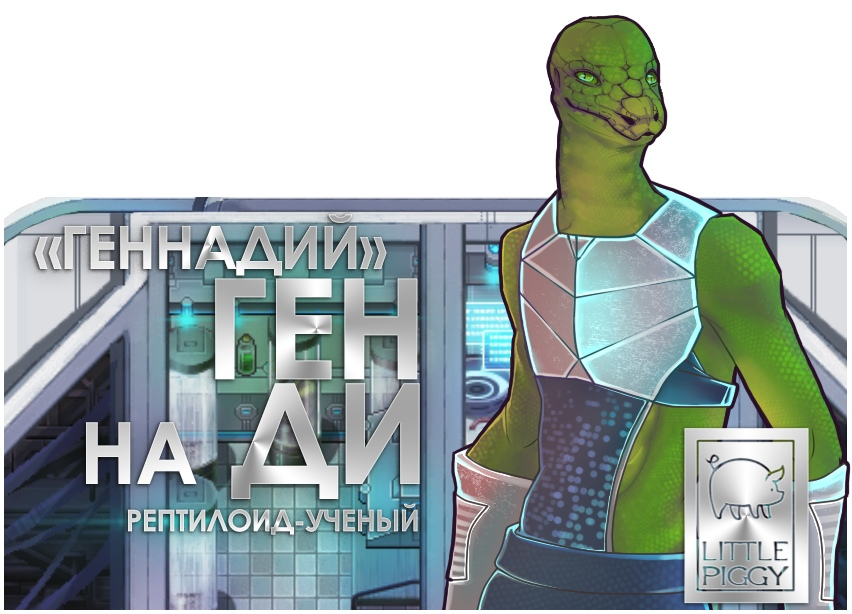# 6 essential JS Game Dev Snippet for rmmv

#### Jonforum

##### VeteranSome cool snippet we absolutely need to put in favorit, with great example.
these basics codes allows you to manage mathematical basis movements in your game. Most essential and basic.

Distance Check
This one is obviously very important in any sort of game development. What I find myself using it for most is quick collision checks. However its used in many cases, an example found later in this article.
PHP:
``````var x = x2 - x1,
y = y2 - y1,
distance = Math.sqrt(x*x + y*y);``````
http://jsfiddle.net/loktar/eYw5b/?utm_source=website&utm_medium=embed&utm_campaign=eYw5b

Move Towards an Object With Easing
This can be useful for inertia based mouse movement. This moves an object to the designated target, making it move slower the closer it gets to the target.
PHP:
``````x += (targetX-x)/speed;
y += (targetY-y)/speed;``````
http://jsfiddle.net/loktar/RE7Ac/?utm_source=website&utm_medium=embed&utm_campaign=RE7Ac

Move Towards an Object at a Constant Speed
The next bit of code can be used for any type of following behavior. The difference from the example above is that the object will move towards its target at a constant speed rather than easing its way there.
PHP:
``````var tx = targetX - x,
ty = targetY - y,
dist = Math.sqrt(tx*tx+ty*ty);
velX = (tx/dist)*thrust;
velY = (ty/dist)*thrust;``````
http://jsfiddle.net/loktar/x5KHT/?utm_source=website&utm_medium=embed&utm_campaign=x5KHT

Get the Angle Between Objects and Project a Point in Front of the Object
Its important to note the above example does not include the facing direction in order to get that we need to know what the angle is between both objects.
PHP:
``````var x = this.x - this.targetX,
y = this.y - this.targetY,
Now that we know how to get the angle between two objects we can project a point in front of it. This can be used for positioning a gun, or for the start position of projectiles. Since realistically bullets shouldn’t necessarily come from the center of our object.
PHP:
``````pointx = x + pointLength * Math.cos(radians);
pointy = y + pointLength * Math.sin(radians);``````
http://jsfiddle.net/loktar/KAa2J/?utm_source=website&utm_medium=embed&utm_campaign=KAa2J

Move Towards Another Object With Proper Rotation
We can combine the two above samples and now move an object towards another with the correct facing angle. This is obviously beneficial for many reasons.
http://jsfiddle.net/loktar/R6RMp/?utm_source=website&utm_medium=embed&utm_campaign=R6RMp

Move an Object Based on its Heading
The last movement example is moving based on the current heading. Meaning moving towards the angle you are facing. The angle can be a target angle, or one set by the user via the arrow keys for example. This is achieved by using the following

PHP:
``````velX = Math.cos(currentAngle) * thrust;
velY = Math.sin(currentAngle) * thrust;``````
However if you want a movement style similar to asteroids you can use this instead.
PHP:
``````velX += Math.cos(currentAngle) * thrust;
velY += Math.sin(currentAngle) * thrust;``````
You will most likely want to set the thrust to a lower number in the above example since you are adding it each time rather than setting it once. One tip if you are using Asteroids style movement is to apply friction or your object will go on forever. This is easily done by doing the following way.
PHP:
``````velX *= 0.98;
velY *= 0.98;``````
Note you can use any value less than one to apply friction the lower the number the faster you will glide to a stop.
Regardless of the type of motion you choose you then apply the velocities to your object.
PHP:
``````this.x -= this.velX;
this.y -= this.velY;``````
And here is a finished example showing the Asteroids style movement.
http://jsfiddle.net/loktar/3M6Fa/?utm_source=website&utm_medium=embed&utm_campaign=3M6Fa

http://www.somethinghitme.com

### Latest Profile Posts

FAST-MAPPING VIDEO

Hey, guys! Do you want to hear a riddle? If so, go to this link!
Omg, 'Marsha' took over the RPGWeb twitter for a bit and its the most adorable thing ever!I like it"Gennady" Gen na Dee reptiloid scientist
I wonder how well Procedural Map generation would work in RPG Maker MZ... Well, only one way to find out! Hehe!